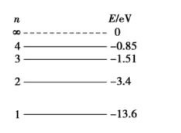$e V$ 的钨时，下列说法中正确的是$\text{A.}$ 氢原子能辐射 4 种不同频率的光子 $\text{B.}$ 氢原子辐射的光子都能使钮发生光电效应 $\text{C.}$ 氢原子辐射一个光子后, 氢原子的核外电子的速率增大 $\text{D.}$ 钨能吸收两个从 $n=4$ 向 $n=2$ 能级跃迁的光子而发生光电效应